# Python - Variables

## Python Variables

In this tutorial, you will learn what are variables in Python and how to use them.

Data items belonging to different data types are stored in computer's memory. Computer's memory locations are having a number or address, internally represented in binary form. Data is also stored in binary form as the computer works on the principle of binary representation. In the following diagram, a string May and a number 18 is shown as stored in memory locations.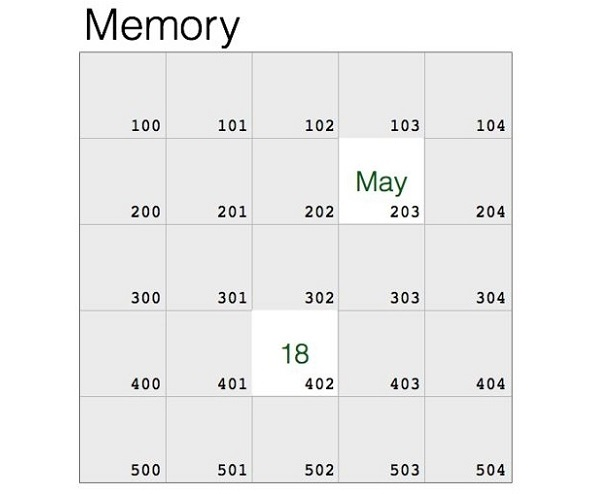If you know the assembly language, you will covert these data items and the memory address, and give a machine language instruction. However, it is not easy for everybody. Language translator such as Python interpreter performs this type of conversion. It stores the object in a randomly chosen memory location. Python's built-in id() function returns the address where the object is stored.

```>>> "May"
>>> id("May")
2167264641264
>>> 18
18
>>> id(18)
140714055169352
```

Once the data is stored in the memory, it should be accessed repeatedly for performing a certain process. Obviously, fetching the data from its ID is cumbersome. High level languages like Python make it possible to give a suitable alias or a label to refer to the memory location.

In the above example, let us label the location of May as month, and location in which 18 is stored as age. Python uses the assignment operator (=) to bind an object with the label.

```>>> month="May"
>>> age=18
```

The data object (May) and its name (month) have the same id(). The id() of 18 and age are also same.

```>>> id(month)
2167264641264
>>> id(age)
140714055169352
```

The label is an identifier. It is usually called as a variable. A Python variable is a symbolic name that is a reference or pointer to an object.

## Python Variables - Naming Convention

Name of the variable is user specified, and is formed by following the rules of forming an identifier.

• Name of Python variable should start with either an alphabet (lower or upper case) or underscore (_). More than one alpha-numeric characters or underscores may follow.

• Use of any keyword as Python variable is not allowed, as keywords have a predefined meaning.

• Name of a variable in Python is case sensitive. As a result, age and Age cannot be used interchangeably.

• You should choose the name of variable that is mnemonic, such that it indicates the purpose. It should not be very short, but not vary lengthy either.

If the name of variable contains multiple words, we should use these naming patterns −

• Camel case − First letter is a lowercase, but first letter of each subsequent word is in uppercase. For example: kmPerHour, pricePerLitre

• Pascal case − First letter of each word is in uppercase. For example: KmPerHour, PricePerLitre

• Snake case − Use single underscore (_) character to separate words. For example: km_per_hour, price_per_litre

Once you use a variable to identify a data object, it can be used repeatedly without its id() value. Here, we have a variables height and width of a rectangle. We can compute the area and perimeter with these variables.

```>>> width=10
>>> height=20
>>> area=width*height
>>> area
200
>>> perimeter=2*(width+height)
>>> perimeter
60
```

Use of variables is especially advantageous when writing scripts or programs. Following script also uses the above variables.

```#! /usr/bin/python3.11
width = 10
height = 20
area = width*height
perimeter = 2*(width+height)
print ("Area = ", area)
print ("Perimeter = ", perimeter)
```

Save the above script with .py extension and execute from command-line. The result would be −

```Area = 200
Perimeter = 60
```

## Python Variables - Assignment Statement

In languages such as C/C++ and Java, one needs to declare the variable and its type before assigning it any value. Such prior declaration of variable is not required in Python.

Python uses = symbol as the assignment operator. Name of the variable identifier appears on the left of = symbol. The expression on its right id evaluated and the value is assigned to the variable. Following are the examples of assignment statements

```>>> counter = 10
>>> counter = 10 # integer assignment
>>> price = 25.50 # float assignment
>>> city = "Hyderabad" # String assignment
>>> subjects = ["Physics", "Maths", "English"] # List assignment
>>> mark_list = {"Rohit":50, "Kiran":60, "Lata":70} # dictionary
assignment
```

Python's built-in print() function displays the value of one or more variables.

```>>> print (counter, price, city)
>>> print (subjects)
['Physics', 'Maths', 'English']
>>> print (mark_list)
{'Rohit': 50, 'Kiran': 60, 'Lata': 70}
```

Value of any expression on the right of = symbol is assigned to the variable on left.

```>>> x = 5
>>> y = 10
>>> z = x+y
```

However, the expression on the left and variable on the right of = operator is not allowed.

```>>> x = 5
>>> y = 10
>>> x+y=z
File "<stdin>", line 1
x+y=z
^^^
SyntaxError: cannot assign to expression here. Maybe you meant '=='
```

Though z=x+y and x+y=z are equivalent in Mathematics, it is not so here. It's because = is an equation symbol, while in Python it is an assignment operator.

## Python Variables - Multiple Assignments

In Python, you can initialize more than one variables in a single statement. In the following case, three variables have same value.

```>>> a=10
>>> b=10
>>> c=10
```

Instead of separate assignments, you can do it in a single assignment statement as follows −

```>>> a=b=c=10
>>> print (a,b,c)
10 10 10
```

In the following case, we have three variables with different values.

```>>> a=10
>>> b=20
>>> c=30
```

These separate assignment statements can be combined in one. You need to give comma separated variable names on left, and comma separated values on the right of = operator.

```>>> a,b,c = 10,20,30
>>> print (a,b,c)
10 20 30
```

The concept of variable works differently in Python than in C/C++.

In C/C++, a variable is a named memory location. If a=10 and also b=10, both are two different memory locations. Let us assume their memory address is 100 and 200 respectively.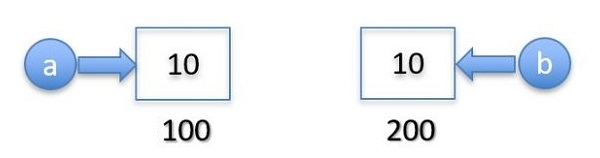If a different value is assigned to "a" - say 50, 10 in the address 100 is overwritten.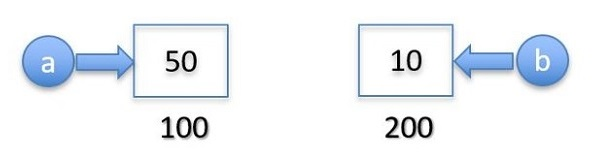A Python variable refers to the object and not the memory location. An object is stored in memory only once. Multiple variables are really the multiple labels to the same object.The statement a=50 creates a new int object 50 in the memory at some other location, leaving the object 10 referred by "b".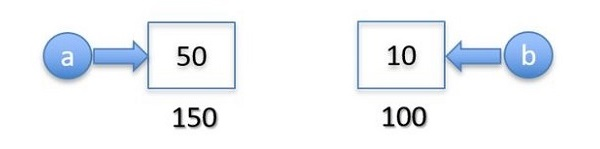Further, if you assign some other value to b, the object 10 remains unreferred.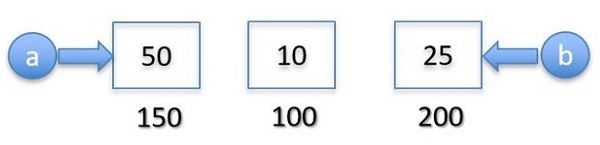Python's garbage collector mechanism releases the memory occupied by any unreferred object.

Python's identity operator is returns True if both the operands have same id() value.

```>>> a=b=10
>>> a is b
True
>>> id(a), id(b)
(140731955278920, 140731955278920)
```